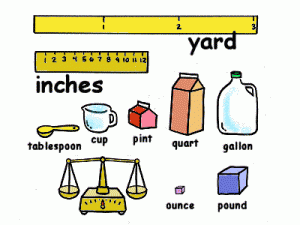# Unit Conversion

This unit conversion guide contains information onstandard units only. The measurements covered here are those used in the metric system and their US equivalents.

### Length or Distance

The international foot ft is equal to 0.3048 m (meter). The inch (in) is equal to 1.0/12.0 ft while the international mile (mi) is 5280.0 ft. The yard (yd) is the same as 3 ft.

The league is an old measuring standard but is sometimes used. It is equal to 3 miles. A furlong is equal to 220 yd while a fathom is equivalent to 6 ft. A rod (rd) is equal to 16.5 ft. The U.S. survey foot (sft) is equal to (1200. /3937.) m.

### Unit Conversion Guide: Mass

A gram (g) is equal to 0.001 kg. The pound mass (lbm) is equivalent to 0.45359237 kg. The carat is the same as 0.2 g. The short ton is equal to 2000 lbm while the long ton is equal to 2240 lbm. An ounce (oz) is equal to 28.34952 g and the grain (gr) is equal to 64.79891 mg. The pennyweight (dwt) is the same as 1.55174 g.

### Force

The pound force (lb) is equal to lbm G while the kilopound kip is the same as 1000 lbf. The kilogram force (kgf) is the same as kg G.

### Unit Conversion Guide: Volume and Area

A gallon (gal) is the same as 3.785412 L (liter). A US pint is equal to 1/8 Gal and a US quart is the same as 2 pint. The US dry pint has as its equivalent 0.5506105 L. A dry US quart is equal to 2 dpint while a hectare is the same as 10000 m^2.

A barrel of petroleum is equal to 158.9873 L and the fluid ounce is the same as 29.57353 mL. The US gill is equal to 0.1182941 L and the tablespoon is the same as 1/32 pint. A teaspoon is the same as 1/3 Tbl.

### Unit Conversion Guide: Energy Conversion

A joule is equal to 1 N m and the British thermal unit (BTU) is equal to 1055.056 J. The calorie is equal to 4.1868 J and the electron volt is the same as 1.602177e-19 J. Erg is the same as 1e-7 J and a ton of TNT is 4.184e9 J.

### Power Conversion

A watt (W) is the same as 1 J/s and a horsepower (hp) is equal to 550 ft lb/s.

### Pressure and Light Conversion

A bar is equal to 1e5 N/m^2 and the Pascal is the same as 1 N/m^2. Pounds per sq. inch is equal to 1 lb/in^2 and pounds per sq. ft. is equivalent to 1 lb/ft^2.

The kilo psi is the same as 1000.0 psi and atmospheres can be converted to 1.01325e5 N/m^2. Inches of Mercury is equal to 3.387 kPa and the Mercury millimeters can be converted to 0.1333 kPa.

A lux is equal to cd/m^2 and the lumen is the same as cd, while the Becquerel is the same as s^-1.

This unit conversion guide is only a brief summary. There are other measurements used in scientific and astronomical studies.